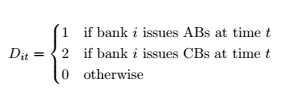## Latex source code - Method 1: latex \documentclass{article} \usepackage{amsmath} \begin{document} \begin{equation} D_{it} = \begin{cases} 1 & \text{if bank $i$ issues ABs at time $t$}\\ 2 & \text{if bank $i$ issues CBs at time $t$}\\ 0 & \text{otherwise} \end{cases} \end{equation} \end{document}- Method 2: latex \Big\{ \begin{matrix} 0 & \text{x>0} \\ 1 & \text{x<=0} \end{matrix}  $\Big\{ \begin{matrix} 0 & x>0 \\ 1 & x<=0 \end{matrix}$ ## Reference - [Multiple lines one side of equation with a Curly Bracket](https://tex.stackexchange.com/questions/337351/multiple-lines-one-side-of-equation-with-a-curly-bracket/337352)